Number ratio (num.ratio) - Dynamic NPU Manual
Select Page

# Number ratio (num.ratio)

Chemical amount

Num.ratio

### Definition in words

Number of component F divided by number of component G in system 1

Note 1: Component B is the quotient of component F and component G.

Note 2: Component F ≠ Component G (≠ means “not equal to”)

Note 3: Component F ∉ Component G (∉ means “is not an element of”)

### Definition by equation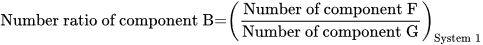### Systematic terms

Number ratio of component B in system 1

### Result type

Ratio scale
• Value sets are positive numerical values
• Algebraic operations are allowed (a=nb; a≠nb)
Numerical values from 0-∞

### Unit

10-6, 10-3, 1 (one), 103, 106, etc.

The unit “1” (one) is ommited in the NPU syntax.

### Reference

Ferard G, Dybkaer R, Fuentes-Arderiu X. Compendium of Terminology and Nomenclature of Properties in Clinical Laboratory Sciences : Recommendations 2016. 1 ed: Royal Society of Chemistry; 2016. 182 p. doi:10.1039/9781782622451.

### Clinical Chemistry

Example 1
Generic abbreviated form

NPU56123 B—Erythroblasts/Leukocytes; num.ratio = ?

Full form with a result
NPU56123 Blood—Erythroblasts/Leukocytes; number ratio = 0.01
Abbreviated form with a result NPU56123 B—Erythroblasts/Leukocytes; num.ratio = 0.01
Written expression The number ratio of Erythroblasts/Leukocytes in Mr. Smith’s blood is [0.01]
Equation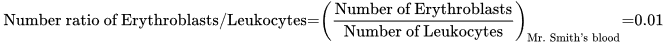Example 2
Generic abbreviated form
NPU58222 B—Neutrophils/Lymphocytes; num.ratio = ?
Full form with a result
NPU58222 Blood—Neutrophils/Lymphocytes; number ratio = 1.65
Abbreviated form with a result NPU58222 B—Neutrophils/Lymphocytes; num.ratio = 1.65
Written expression The number ratio of Neutrophils/Lymphocytes in Mr. Smith’s blood is [1.65]
Equation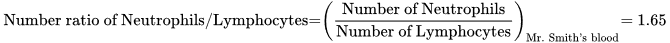### Clinical Immunology

Example 3
Generic abbreviated form
NPU21856 Lymcs(B)—T-lymphocytes(helper/cytotox); number ratio = ?
Full form with a result
NPU21856 Lymphocytes(Blood)—T-lymphocytes(helper/cytotox); number ratio = ?
Abbreviated form with a result
NPU21856 Lymcs(B)—T-lymphocytes(helper/cytotox); number ratio = ?
Written expression The number ratio of T-lymphocytes(helper/cytotox) in Mr. Smith’s blood is [?]
Equation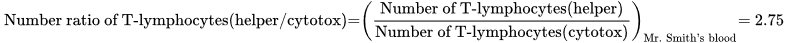Example 4
Generic abbreviated form
NPU56977 Lymcs(BAL)—T-lymphocytes(helper/cytotox); number ratio = ?
Full form with a result
NPU56977 Lymphocytes(Bronchoalveolar Lavage Fluid)—T-lymphocytes(helper/cytotox); number ratio = ?
Abbreviated form with a result NPU56977 Lymcs(BAL)—T-lymphocytes(helper/cytotox); number ratio = ?
Written expression The number ratio of T-lymphocytes(helper/cytotox) in Mr. Smith’s Bronchoalveolar Lavage Fluid is [?]
Equation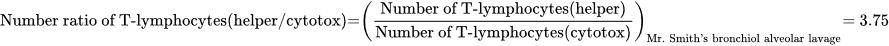### Reference

NPU database, https://www.ifcc.org/ifcc-scientific-division/sd-committees/c-npu/npusearch/ and http://www.labterm.dk/default.aspx
• # Kind-of-property

• ## Kind-of-quantity

### Reference

• Dybkaer R. Concept system on ‘quantity’: formation and terminology. Accredit Qual Assur. 2013;18(3):253-60.
• Dybkaer R. ISO terminological analysis of the VIM3 concepts ‘quantity’ and ‘kind-of-quantity’. Metrologia. 2010;47(3):127-34.
Date Term Note
1996-01-01 Number ratio Term established

QU71685